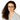# Probabilistic Programming and Bayesian Inference in Python

120-minute Tutorial - Sunday, July 28 at 1:15pm in Suzanne Scharer

If you can write a model in sklearn, you can make the leap to Bayesian inference with PyMC3, a user-friendly intro to probabilistic programming (PP) in Python. PP just means building models where the building blocks are probability distributions! And we can use PP to do Bayesian inference easily. Bayesian inference allows us to solve problems that aren't otherwise tractable with classical methods.

Let's build up our knowledge of probabilistic programming and Bayesian inference! All you need to start is basic knowledge of linear regression; familiarity with running a model of any type in Python is helpful.

By the end of this presentation, you'll know the following: - What probabilistic programming is and why it's necessary for Bayesian inference - What Bayesian inference is, how it's different from classical frequentist inference, and why it's becoming so relevant for applied data science in the real world - How to write your own Bayesian models in the Python library PyMC3, including metrics for judging how well the model is performing - How to go about learning more about the topic of Bayesian inference and how to bring it to your current data science job

We'll meet our objectives by answering three questions:

1. What is probabilistic programming?

• PP is the idea that we can use computer code to build probability distributions
• Theory of the primitives in probabilistic programming and how we can build models out of distributions
2. What is Bayesian inference and why should I add it to my toolbox on top of classical ML models?

• Classically, we had simulations, but they run in only one direction: get data input and move it according to assumptions of parameters and get a prediction
• Bayesian inference adds another direction: use the data to go back and pick one of many possible parameters as the most likely to have created the data (posterior distributions)
• Use Bayes' theorem to find the most likely values of the model parameters
3. What is PyMC3 and how can I start building and interpreting models using it?

• We'll work through actual examples of models using PyMC3, including hierarchical models
• Solving Bayes’ theorem in practice requires taking integrals
• If we don’t want to do integrals by hand, we need to use numerical solution methods
• From the package authors: "[PyMC3 is an ]open source probabilistic programming framework written in Python that uses Theano to compute gradients via automatic differentiation as well as compile probabilistic programs on-the-fly to C for increased speed"

The intention is to get hands-on experience building PyMC3 models to demystify probabilistic programming / Bayesian inference for those more well versed in traditional ML, and, most importantly, to understand how these models can be relevant in our daily work as data scientists in business.

## Prerequisites & Setup Instructions

Participants should have Jupyter notebooks set up with an environment that has PyMC3 installed. They can run it in a base environment, but having a dedicated PyMC3 environment is preferred as that package tends not to play nicely with others. I would be running an interactive session so participants should be able to run the notebook along with the tutorial.

## Presented by:Lara Kattan